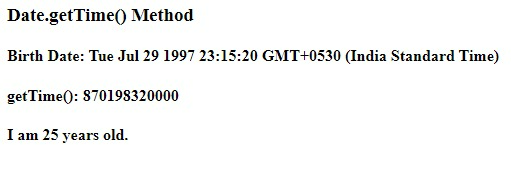GFG App
Open AppBrowser
Continue

# JavaScript Date getTime() Method

JavaScript Date getTime() Method is used to return the number of milliseconds since 1 January 1970. when a new Date object is created it stores the date and time data when it is created. When the getTime() method is called on this date object it returns the number of milliseconds since 1 January 1970 (Unix Epoch). The getTime() always uses UTC for time representation.

Syntax:

`Date.getTime()`

Parameters: This method does not accept any parameter.

Return type: A numeric value equal to no of milliseconds since Unix Epoch.

Below are examples of the Date getTime() Method:

Example 1:

## Javascript

 `// Here a date has been assigned ` `// While creating Date object ` `let A = ``new` `Date(``'October 15, 1996 05:35:32'``); `   `// Hour from above is being ` `// extracted using getTime() ` `let B = A.getTime(); `   `// Printing time in milliseconds. ` `console.log(B);`

Output:

`845337932000`

Example 2: Here, the date of the month must lie between 1 to 31 because no date can have a month greater than 31. That is why it returns NaN i.e, Not a Number if the month in the Date object is greater than 31. Hours will not have existed when the date of the month is given as 33 i.e, greater than 31.

## Javascript

 `// Creating a Date object ` `let A = ``new` `Date(``'October 35, 1996 12:35:32'``); ` `let B = A.getTime();`   `// Printing hour. ` `console.log(B);`

Output:

`NaN`

Example 3: Here, we will calculate the user’s age by providing the birth date of the user.

## HTML

 `` `<``html` `lang``=``"en"``>` `<``head``>` `    ``<``title``>JavaScript Date getTime() Method` `` `<``body``>` `    ``<``h3``>Date.getTime() Method` `    ``<``div``>` `        ``<``h4``>Birth Date:` `            ``<``span` `class``=``"date"``>` `        ``` `    ``` `    ``<``div``>` `        ``<``h4``>getTime():` `            ``<``span` `class``=``"time"``>` `        ``` `    ``` `    ``<``div``>` `        ``<``h4``>I am` `            ``<``span` `class``=``"years"``>` `                ``years old.` `        ``` `    ``` `    ``<``script``>` `        ``let BD = new Date("July 29, 1997 23:15:20");` `        ``let date = document.querySelector(".date");` `        ``let time = document.querySelector(".time");` `        ``let Today = new Date();` `        ``let today = Today.getTime();` `        ``let bd = BD.getTime();` `        ``let year = 1000 * 60 * 60 * 24 * 365;` `        ``let years = (today - bd) / year;` `        ``date.innerHTML = BD;` `        ``time.innerHTML = BD.getTime();` `        ``let y = document.querySelector(".years");` `        ``y.innerHTML = Math.round(years);` `    ``` `` ``

Output:Supported Browsers: The browsers supported by JavaScript Date getTime() method are listed below: